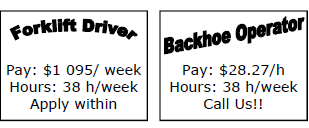Fractions, decimals and percentages
Financial Maths
Indices
Numbers of any magnitude
Algebra
100

Convert 70% to a fraction.

7/10

100

Find John's daily pay if he work 7hours a day at \$15 an hour.

\$105

100

Evaluate 5

125

100

What does the prefix KILO stand for ?

1 thousand

100

Multiply x and 5y and to this result add 9

5xy + 9

200

Convert the following percentages to a decimal 63.2%

0.632

200

Rami earns \$28.20 per hour. He works 7 hours one day and 5 hours the next day. How much money did he earn in two days?

\$338.4

200

Simplify 2x 25

28

200

127 x 43 = round to the nearest hundredth

5,500

200

x = 2, y = 3 and z = 4a

x + 2y + 3z =

20

300

What is 19% of \$84

\$15.96

300

Jen earns \$567 a week what is her yearly Salary?

\$29,484

300

(2x 39)  ÷  (2x 34)

22 x 35

300

Round to 2 d.p 27.36567

27.37

300

Solve 7(x+3) - 2 = 33

x = 2

400

What percentage is 28min of 3 hours (round to 2 d.p)?

15.56%

400

John invests \$6000. He receives 4% of his investment per year as interest. How much interest doest he have after 5 years?

\$1200

400

(24 x 34) ÷ (?? x ?) = 23 x 32

2 x 32

400

4 centuries = how many decades

40

400

(8a)2 ÷ 16a =

4a

500

Increase 50 by 15%.

57.5

500Which job is better?

Fortlift Driver

500

(23 x 34 )2

2x 38

500

What is the smallest prefix?

yocto

500

If C = 59(F – 32), find C when F = 212

10620

Click to zoom